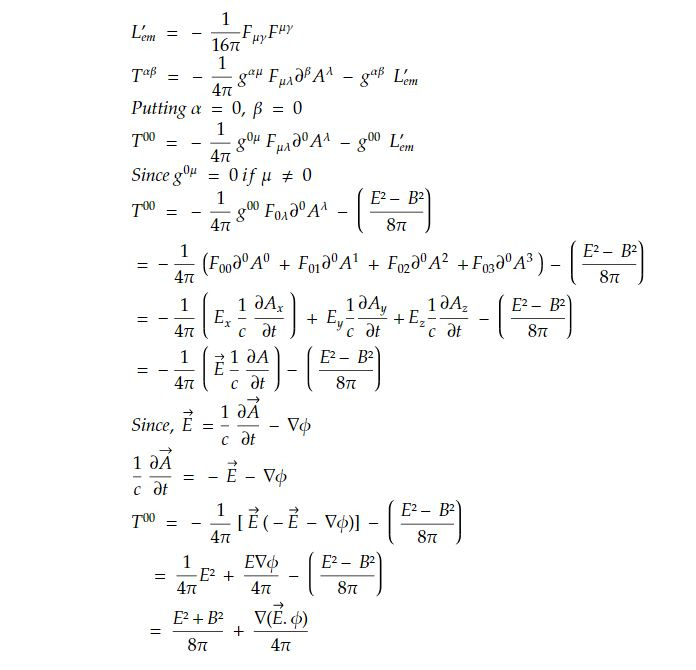# Canonical stress tensor

The canonical stress tensor is a concept in theoretical physics, particularly in the context of classical field theory and quantum field theory. It is used to describe the distribution of momentum and stress within a physical system. The canonical stress tensor has different forms in classical and quantum field theory:

1. Classical Field Theory: In classical field theory, such as classical electrodynamics or classical fluid dynamics, the canonical stress tensor represents the momentum and stress associated with a continuous field (e.g., electromagnetic field or fluid velocity field). It is defined as:

T_ij = ϵ₀(E_iE_j + B_iB_j) - (1/2)δ_ij(ϵ₀E^2 + (1/μ₀)B^2) + (1/μ₀)(E_iB_j + E_j*B_i)

Where:

• T_ij is the canonical stress tensor.

• E_i and B_i are the components of the electric and magnetic fields, respectively.

• ϵ₀ is the vacuum permittivity.

• μ₀ is the vacuum permeability.

• δ_ij is the Kronecker delta.

This tensor describes the distribution of electromagnetic momentum and stress in the electromagnetic field. It is useful for understanding the forces and pressures associated with electromagnetic fields in classical physics.

2. Quantum Field Theory: In quantum field theory, the canonical stress tensor serves a similar purpose but is adapted to the framework of quantum mechanics. It is used to describe the distribution of energy, momentum, and stress in a quantum field. In quantum electrodynamics, for instance, the canonical stress-energy-momentum tensor for the electromagnetic field is defined as:

**T^(μν) = (1/4π) [(F^(μλ)F_λ^ν - (1/4)g^(μν)F^(αβ)F_αβ)]

Where:

• T^(μν) is the canonical stress-energy-momentum tensor.

• F^(μν) is the electromagnetic field tensor.

• g^(μν) is the metric tensor in spacetime.

This tensor describes how energy, momentum, and stress are distributed in the electromagnetic field in the context of quantum physics. It plays a crucial role in understanding quantum electrodynamics and other quantum field theories.

For transition from Lagrangian to Hamiltonian density , we defined

The Hamiltonian of a systemThe Hamiltonian of a system isIf it is not explicit function of time

∂H/∂t = 0

And if qi is cyclic coordinateWhen we go from particle to field description, Hamiltonian for particle is replaced by Hamiltonian density so that

Total Hamiltonian

H = ∫ H' d³x dt

d³x dt - > 4 dimensional volume element

Similarly, q -> 𝜙_k,So the Lagrangian density L' is function of 𝜙_k and ∂^β𝜙_k

The generalized momentum for fieldAnd Hamiltonian densityFor electromagnetic field,

L'= L'emThe covariant form of H' isFor free electromagnetic field,= energy density + added term

The canonical stress tensor has several important uses in the fields of classical and quantum physics, particularly in understanding the distribution of momentum, energy, and stress in physical systems. Here are some of its useful applications:

1. Electromagnetic Forces and Pressure: In classical electrodynamics, the canonical stress tensor helps calculate the electromagnetic forces and pressures acting on charged particles and conductors. This is essential in designing electromagnetic devices and understanding how electric and magnetic fields interact with matter.

2. Stress in Materials: In solid-state physics and materials science, the canonical stress tensor is used to study the stress and strain distributions in materials under various conditions. This is important for predicting material behavior under stress, designing structures, and understanding mechanical properties.

3. Fluid Dynamics: In classical fluid dynamics, the canonical stress tensor is adapted to describe the momentum and stress distribution in a fluid. It helps analyze fluid flow, pressure, and the forces acting on submerged objects, making it valuable in engineering applications.

4. Quantum Field Theory: In quantum field theory, the canonical stress-energy-momentum tensor is crucial for understanding the behavior of quantum fields. It plays a central role in the formulation of quantum field equations, such as the Klein-Gordon equation, Dirac equation, and Maxwell's equations in a quantum context.

5. General Relativity: In the context of general relativity, the canonical stress-energy-momentum tensor is used to describe the distribution of energy and momentum in spacetime. It plays a fundamental role in Einstein's field equations, which describe the gravitational interaction between matter and spacetime curvature.

6. Quantum Electrodynamics: In quantum electrodynamics (QED), the canonical stress-energy-momentum tensor for the electromagnetic field is used to understand the behavior of photons and charged particles in quantum interactions. QED is a foundational theory for understanding electromagnetism at the quantum level.

7. Cosmology: In cosmology, the canonical stress-energy-momentum tensor is used to model the distribution of energy and momentum in the universe. It helps cosmologists study the dynamics of the cosmos and make predictions about its evolution.

8. Experimental Predictions: The canonical stress tensor's theoretical calculations can lead to predictions that can be tested experimentally. For example, it can be used to predict the behaviour of particles and fields in particle accelerators or the behaviour of materials under various conditions.

Overall, the canonical stress tensor is a versatile tool that finds applications across a wide range of physical disciplines. It enables physicists and engineers to analyze and predict the behaviour of physical systems, from electromagnetic interactions to material properties and the dynamics of the universe.

This note is a part of the Physics Repository.